﻿ Turn A Square: generative aRt – Data Science Austria

# Turn A Square: generative aRt

A while back I visited Artistes & Robots in Paris. Part of the exhibition was on the origins of computer-based art. Nowadays this is referred to as generative art, where computers generate artwork according to rules specified by the programmer. I wanted to emulate some of the early generative artwork I saw there, using R.

Some examples of early generative art

Georg Nees was a pioneer of computer-based artwork and he has a series of pieces using squares. An example I found on the web was Schotter (1968).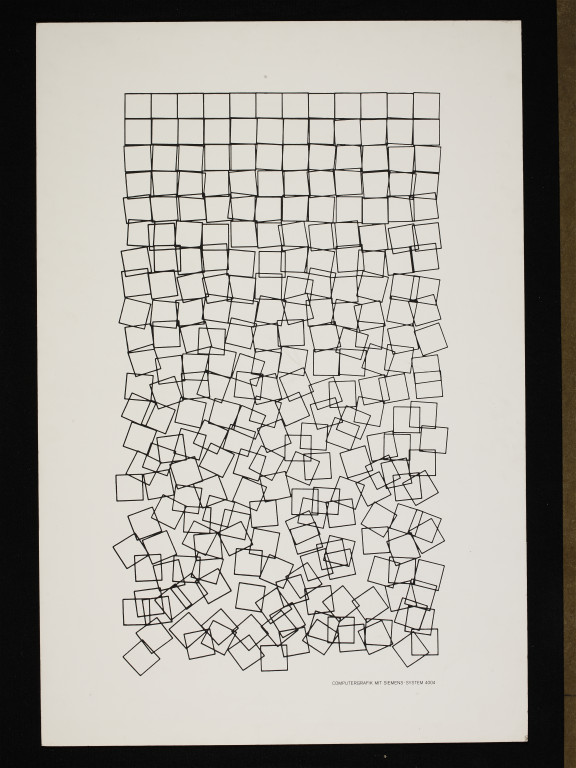CIS:E.217-2008

Another example using rotation of squares is Boxes by William J. Kolomyjec.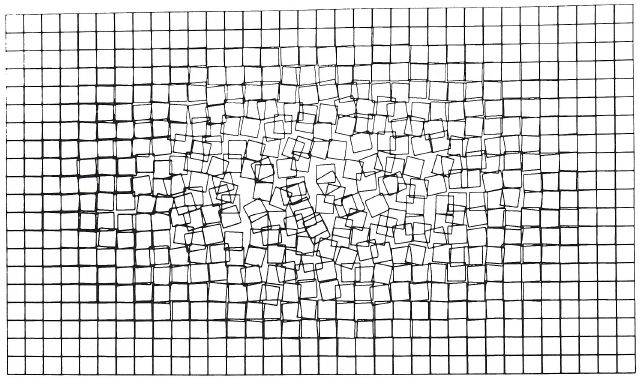Boxes

I set out to generate similar images where the parameters can be tweaked to adjust the final result. The code is available on GitHub. Here is a typical image. Read on for an explanation of the code.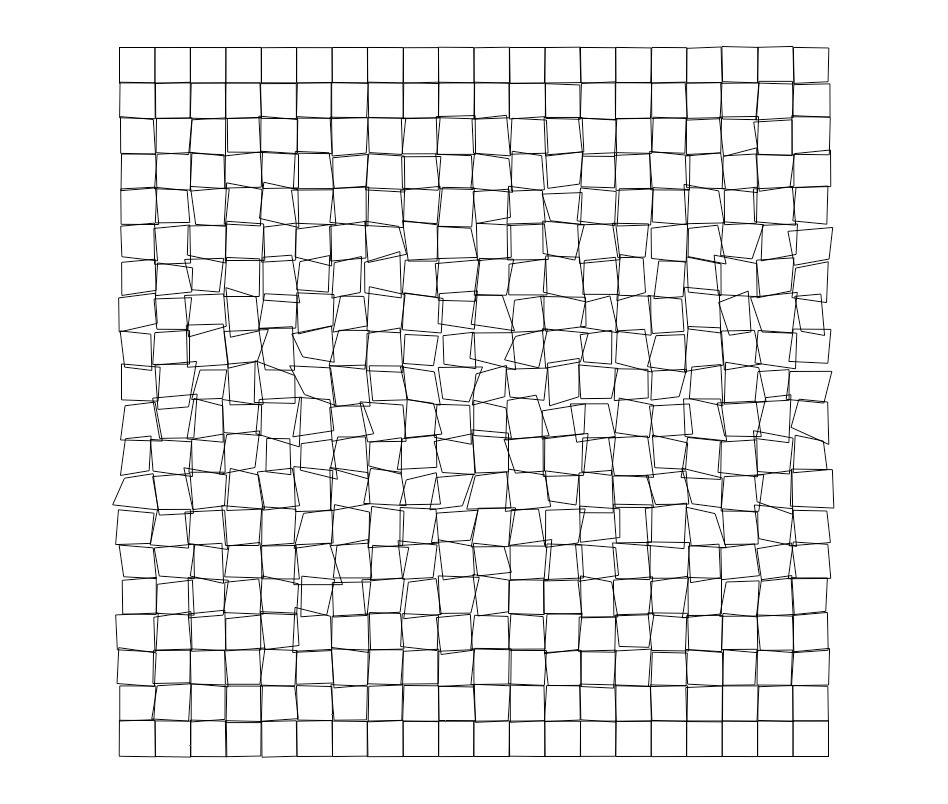Generating a grid of squares

I started by generating a grid of squares. We can use the segment command in R to do the drawing.

```xWave <- seq.int(1:10)
yWave <- seq.int(1:10) for (i in seq_along(xWave)) { xCentre <- xWave[i] for (j in seq_along(yWave)) { yCentre <- yWave[j] lt <- c(xCentre - 0.4,yCentre - 0.4) rt <- c(xCentre + 0.4,yCentre - 0.4) rb <- c(xCentre + 0.4,yCentre + 0.4) lb <- c(xCentre - 0.4,yCentre + 0.4) new_shape_start <- rbind(lt,rt,rb,lb) new_shape_end <- rbind(rt,rb,lb,lt) new_shape <- cbind(new_shape_start,new_shape_end) if(i == 1 && j == 1) { multiple_segments <- new_shape } else { multiple_segments <- rbind(multiple_segments,new_shape) } }
}
par(pty="s")
plot(0, 0, xlim=c(min(xWave)-1,max(xWave)+1), ylim=c(min(yWave)-1,max(yWave)+1), col = "white", xlab = "", ylab = "", axes=F) segments(x0 = multiple_segments[,1], y0 = multiple_segments[,2], x1 = multiple_segments[,3], y1 = multiple_segments[,4])
```A simple grid

We’re using base R graphics to make this artwork – nothing fancy. Probably the code can be simplified!

The next step was to add some complexity and flexibility. I wanted to be able to control three things:

1. the size of the grid
2. the grout (distance between squares)
3. the amount of hysteresis (distorting the squares, rather than rotating them).

Here is the code. See below for some explanation and examples.

```# this function will make grid art
# arguments define the number of squares in each dimension (xSize, ySize)
# grout defines the gap between squares (none = 0, max = 1)
# hFactor defines the amount of hysteresis (none = 0, max = 1, moderate = 10)
make_grid_art <- function(xSize, ySize, grout, hFactor) { xWave <- seq.int(1:xSize) yWave <- seq.int(1:ySize) axMin <- min(min(xWave) - 1,min(yWave) - 1) axMax <- max(max(xWave) + 1,max(yWave) + 1) nSquares <- length(xWave) * length(yWave) x <- 0 halfGrout <- (1 - grout) / 2 for (i in seq_along(yWave)) { yCentre <- yWave[i] for (j in seq_along(xWave)) { if(hFactor < 1) { hyst <- rnorm(8, halfGrout, 0) } else { hyst <- rnorm(8, halfGrout, sin(x / (nSquares - 1) * pi) / hFactor) } xCentre <- xWave[j] lt <- c(xCentre - hyst,yCentre - hyst) rt <- c(xCentre + hyst,yCentre - hyst) rb <- c(xCentre + hyst,yCentre + hyst) lb <- c(xCentre - hyst,yCentre + hyst) new_shape_start <- rbind(lt,rt,rb,lb) new_shape_end <- rbind(rt,rb,lb,lt) new_shape <- cbind(new_shape_start,new_shape_end) if(i == 1 && j == 1) { multiple_segments <- new_shape } else { multiple_segments <- rbind(multiple_segments,new_shape) } x <- x + 1 } } par(mar = c(0,0,0,0)) plot(0, 0, xlim=c(axMin,axMax), ylim=c(axMax,axMin), col = "white", xlab = "", ylab = "", axes=F, asp = 1) segments(x0 = multiple_segments[,1], y0 = multiple_segments[,2], x1 = multiple_segments[,3], y1 = multiple_segments[,4])
}
```

The amount of hysteresis is an important element. It determines the degree of distortion for each square. The distortion is done by introducing noise into the coordinates for each square that we map onto the grid. The algorithm used for the distortion is based on a half-cycle of a sine wave. It starts and finishes on zero distortion of the coordinates. In between it rises and falls symmetrically introducing more and then less distortion as we traverse the grid.

Changing the line of code that assigns a value to hyst will therefore change the artwork. Let’s look at some examples.

```# square grid with minimal hysteresis
make_grid_art(10,10,0.2,50)
``````# square grid (more squares) more hysteresis
make_grid_art(20,20,0.2,10)
``````# rectangular grid same hysteresis
make_grid_art(25,15,0.2,10)
``````# same grid with no hysteresis
make_grid_art(25,15,0.2,0)
```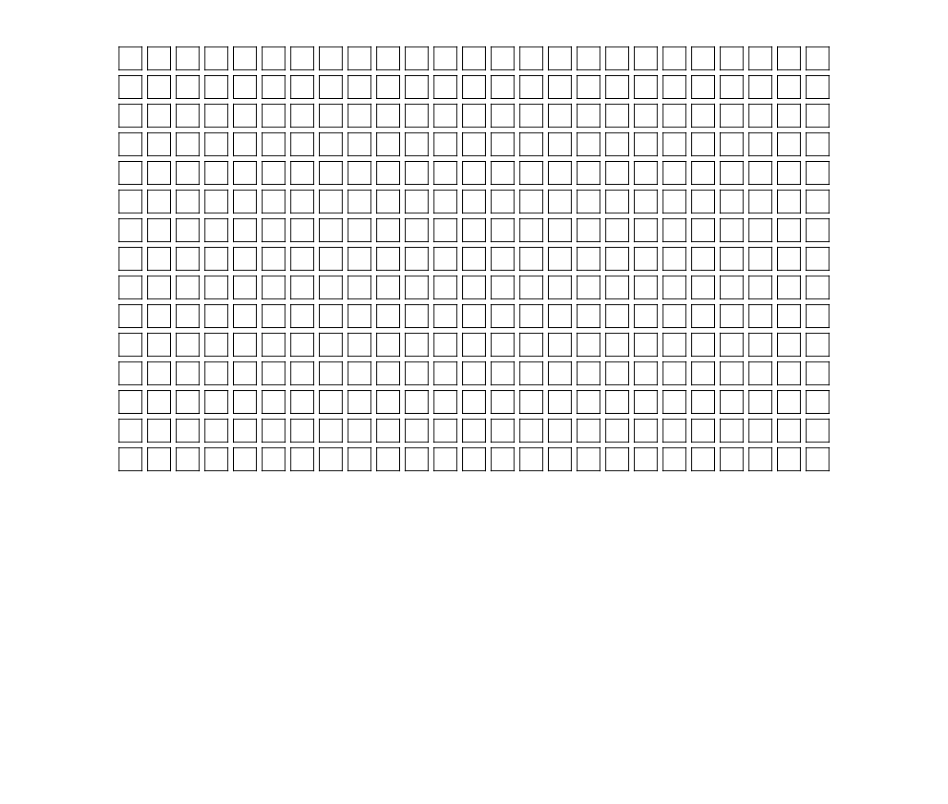```# square grid moderate hysteresis and no grout
make_grid_art(20,20,0,10)
```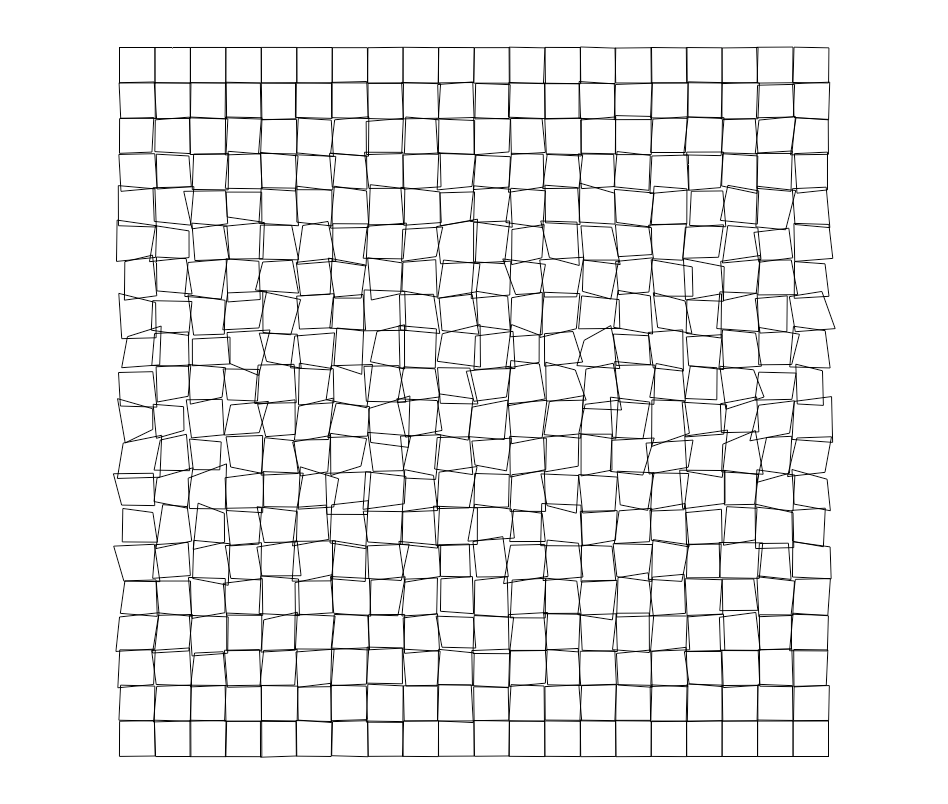Hopefully this will give you some ideas for simple schemes to generate artwork in R. I plan to add to the GitHub repo when get the urge. There is already some other generative artwork code in there for Igor Pro. Two examples are shown below (I don’t think I have written about this before on quantixed).

The post title comes from “Turn A Square” by The Shins from their album Chutes Too Narrow.